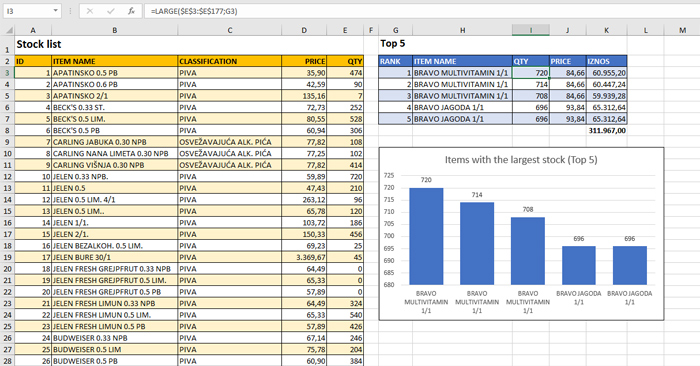# Items with the largest stocks

The business of most companies is impossible without keeping a certain level of inventory. Excess items in stock, if there is no economic justification for it, is a loss that is reflected, both through the cost of storage and through the amount of captured working capital. Excel has a very simple way to determine the quantity of items that are most in stock, as well as any additional information regarding those items.

Stock data is stored in the stock list, which is a table that contains all the necessary data on items as well as quantities in stock. To determine the 5 items with the highest stock level, we will make an additional table in which we first list the RANK column, with values ​​from 1 to 5. Then we add the QTY column in which we will calculate the highest stock level, which we do using the formula:

=LARGE(\$E\$3:\$E\$177,G3)

The first argument is a quantity column (QTY), and the second is a parameter from the RANK column. If we copy the formula into the remaining cells, we get the quantities of the 5 items that are most in stock. In a similar way we could calculate the lowest stocks; we would only use the SMALL function instead of LARGE, but that is not the subject of this post, so let’s avoid additional details.

How to determine which items are these? For this we will use the LOOKUP function where, based on the given stock level, we search for the appropriate columns. To find the name of the item, we will write the formula:

=LOOKUP(I3,\$E\$3:\$E\$177,\$B\$3:\$B\$177)

And the associated prices are found using the formula:

=LOOKUP(I3,\$E\$3:\$E\$177,\$D\$3:\$D\$177)

Further, it is possible to calculate the amounts, the total amount, as well to make a simple chart showing the items with the largest stocks.